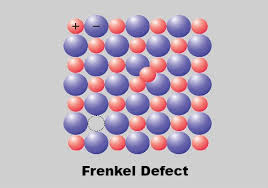## How to Calculate and Solve for Frenkel Defect | CeramicsThe image above represents frenkel defect.

To compute for frenkel defect, four essential parameters are needed and these parameters are N, activation energy (Qfr), Boltzmann’s Constant (K) and temperature (T).

The formula for calculating the frenkel defect:

Nfr = N exp (-Qfr / 2KT)

Where:

Nfr = Frenkel Defect
Qfr = Activation Energy
K = Boltzmann’s Constant
T = Temperature

Let’s solve an example;
Find the frenkel defect when the activation energy is 34, N is 22, Temperature is 12 and the boltzmann’s constant is 1.38064852e-23.

This implies that;

Qfr = Activation Energy
K = Boltzmann’s Constant
T = Temperature

Nfr = N exp (-Qfr / 2KT)
Nfr = (22)exp(-(34) / 2(1.38064852e-23)(14))
Nfr = (22)exp((-34) / (3.865815856e-22))
Nfr = (22)exp(-8.795038684325786e+22)
Nfr = (22)(0)
Nfr = 0

Therefore, the frenkel defect is 0.

Calculating the Activation Energy when the Frenkel Defect, the Boltzmann’s Constant and the Temperature is Given.

Qfr = – (In (Nfr / N) x 2KT)

Where;

Qfr = Activation Energy
Nfr = Frenkel Defect
K = Boltzmann’s Constant
T = Temperature

Let’s solve an example;
Given that the frenkel defect is 20, the boltzmann’s constant is 5, the temperature is 2 and N is 10. Find the activation energy?

This implies that;

Nfr = Frenkel Defect = 20
K = Boltzmann’s Constant = 5
T = Temperature = 2
N = 10

Qfr = – (In (Nfr / N) x 2KT)
Qfr = – (In (20 / 10) x 2 x 5 x 2)
Qfr = – (In 2 x 20)
Qfr = – (In 40)
Qfr = – 3.688

Therefore, the activation energy is – 3.688.